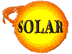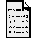How is SOHO Orbit Location (The L1 point) Calculated?(by Amara Graps)

SOHO has an uninterrupted view of the Sun.

That view of the Sun is achieved by operating SOHO from a permanent vantage point 1.5 million kilometers (about 4 times as far away as the moon) ahead of the Earth in a "halo orbit" around the L1 Lagrangian point, where it has a pointing accuracy of 10". All previous solar observatories have orbited the Earth, where their observations were periodically interrupted as our planet `eclipsed' the Sun.

Look at an orbital schematic for SOHO's halo orbit.

The halo orbit has a period of 180 days. The semi-diameters of the halo orbit have typical dimensions of approximately 200,000 km in the ecliptic in the Earth-Sun direction, 650,000 km in the ecliptic perpendicular to the Earth-Sun direction, and 200,000 km out of the ecliptic.

The L1 halo orbit has several particular advantages as compared to low Earth orbits:

• It provides a smooth Sun-spacecraft velocity change throughout the orbit, appropriate for helioseismology.
• It is permanently outside of the magnetosphere, appropriate for the ``in situ'' sampling of the solar wind and particles.
• It allows permanent observation of the Sun, appropriate for all the investigations.

You may jump to Goddard's Exploration WWW Site for a nice intuitive, elementary description about the Lagrangian points.

The calculation of Lagrange points can be found in books on orbital or celestial mechanics. One of my favorites is:

Fundamentals of Celestial Mechanics by J.M.A. Danby, Willmann-Bell, 1988.
In particular, look at your celestial mechanics textbook under the category of the Three-Body Problem, usually something like "Positions of Equilibrium" or "The Libration Points." (In the above text, this subject is in Chapter 8).

Also check out:

(Information from the Danby book and the site mentioned above.)

The general problem of the motion of three bodies (assumed to be point masses) subject only to their mutual gravitational attractions has not been solved analytically. Think about this. It's only slightly more complicated than the two-body problem! Nevertheless, if one imposes some special constraints on the three-body problem (the so-called "restricted three-body problem"), some particular solutions can be derived, and it has important applications to actual celestial objects (consider the Sun-Earth-Moon system or a Saturn-moonlet-ring particle system).

The restricted three-body problem is where two bodies of a finite mass revolve around one another and a third body of infinitesimal mass moves in their field. The third object essentially is unable to measurably affect the motion of the two other bodies. Since the orbits of the two finite mass bodies conform to the known solution of the two-body case, the problem is reduced to the study of the motion of only the small test particle in the field of the two coorbiting primaries. In order to simplify the problem even further, we can consider the *circular* restricted three-body problem, in which the two finite masses are assumed to move in circular orbits about one another. There is also the *elliptic* restricted three-body problem, which is the topic of considerable research activity.

If one sets up the circular restricted three body problem so that the center of mass of the two finite objects is the origin and the axes rotate with constant angular velocity, the first thing to fall out of one of the constants of integration is an energy integral called "Jacobi's integral" (after the German mathematician Carl Gustav Jacob Jacobi), and it represents the conservation of energy in the rotating system. Five additional integrals are required for the restricted three body problem's complete solution, but none has ever been found, even though people have cleverly tried to invoke things like conservation of angular momentum to get a second integral.

So assume that we now have the Jacoby Integral. We want to know the positions of equilibrium of this system.

There exist five fixed points in the CR3BP (Circular Restricted Three-Body Problem). Also known as Lagrangian, or Libration points, these points represent points in space where an unmoving particle would remain for all time. These fixed points are all points where all six phase variables time derivatives are zero. In other words, the libration points, are solutions to the equations of motion for the spacecraft such that the x, y, and z positions and x, y, and z velocities are all 0.

To compute the location of these points, one must solve the equations of motion under these special conditions. When solving, one finds that there are 5 solutions to these equations:

• Two solutions fall out easily; each forms and equilateral triangle with the Earth and the Sun in the plane of rotation.
• Each of the 3 remaining solutions also lie in the plane of rotation, on the line between the Earth and Sun.

Two of these points, known as L4 and L5 form equilateral triangles with the primary masses. The other three libration points (L1, L2, L3) are colinear with the primary masses, and are found by solving quintic polynomials dictated by the equations of motion.

These solutions are diagrammed at the Geometry Web Site.

To find the location of the 3 libration points lying on the Earth-Sun axis (L1, L2, and L3), one must solve for the x coordinate of each. This boils down to solving a quintic polynomial equation.

The polynomial equation is the following.

Let V be a modified potential of this CR3BP system:

-V = (1/2)(x^2 + y^2) + (1-mu)/r1 + mu/r2 (1)
where mu is the ratio of the smaller mass to that of the larger mass (3.03591e-6 for the Earth-Sun system) r1 and r2 are the distances from the massless particle to mass1 and mass2. The surface of zero relative velocity is
x^2 + y^2 + 2*(1-mu)/r1 + 2*mu/r2 = Constant (2)
The surface of zero relative velocity is given by the function:
F(x,y,z) = V + 1/2*Constant = 0. (3)

There are two locations of equilibrium that a particle will move to, if given a nudge. You can see graphics of the two stable points here.

So let's say a particle is given a nudge. It will go to one of two locations. The condition for the two places is:

dF/dx = dF/dy = dF/dz = 0 (4)
(all "d"s are partial derivatives)
and we have from our relationship for our modified potential (1) and the function F: (3)
dF/dz = z((1-mu)/r1^3 + mu/r2^3) (5)
Any double point in the xy plane is where z = 0, so using the conditions for dF/dx=0 and dF/dy=0 (equations 4) we get
x - (1-mu)*(x-x1)/r1^3 - mu*(x-x2)/r2^3 = 0 (6)
y - (1-mu)*y/r1^3 - mu*y/r2^3 = 0 (7)

Equation (7) is satisfied if y = 0 (Remember we are seeking a quintic polynomial along the x-line) and so equation (6) becomes:
x - (1-mu) * (x-x1)/ |x-x1|^3 - mu*(x-x2)/ |x-x2|^3 = 0 (8)***
where | | indicates a positive value of the difference.

There are 3 real roots of the above equation along the x-axis. These correspond to the Lagrangian points L1, L2, and L3. Remember that around L1 is where SOHO is located in a Halo orbit. (If you would like to continue this exercise to find the Lagrangian L4 and L5 positions, then set y not equal to 0 in equation (7).)

The Geometry Web page expands Equation (8) out for L1 into:

-((1-mu)(1-mu+x)^2) + mu(mu+x)^2 + x(1-mu+x)^2(mu+x)^2 = 0

And you can see curves for the equations for L1 corresponding quintic polynomials for several values of mu.

Now what about the Halo Orbits?

Once the Libration points have been located, one can search for orbits about these points.

Surrounding each of the three collinear libration points (L1, L2, L3) exist families of periodic orbits. These periodic orbits, known as "halo orbits", provide excellent outposts for astronomical observation. So if we look out at one of the libration points, say the L1 point, we would find the spacecraft orbiting it in a small circle that resembles a halo around L1. Halo orbits' proximity to the Earth, and accesiblity to low-velocity space craft provide an economical opportunity to expand out knowledge about our solar system. Furthermore, the existence of stable and unstable manifolds provide cheap transport of space craft to these periodic orbits.

From SOHO's L1 halo orbit, the Sun is always visible, so the spacecraft is spared the hiding of the sun that happens for spacecraft in orbit around the Earth. Because its launch was so accurate, enough fuel remains on board to keep it in its position for 20 years, far longer than the original planned 6 years.

The mathematics of the halo orbit is quite complicated, the material of which one would find in advanced orbital mechanics courses. But the Geometry Center at the University of Minnesota has put together a beautiful set of WWW pages with diagrams and equations to show how to find the halo orbits, how to analyze their stability and how to compute the stable and unstable manifolds of halo orbits.

For more detailed information, consult the Halo Orbits of the Circular Restricted Three Body Problem web site.

```

```
Material also drawn from the SOHO Web site http://sohowww.nascom.nasa.gov and "The SOHO Mission: An Overview", by V. Domingo et al., _The SOHO Mission_ ed B. Fleck, V. Domingo, and A. Poland, Kluwer Press, 1995, pg. 28.

Back to the FAQsBack to the SOLAR CenterComments?

Special Thanks to A. Graps